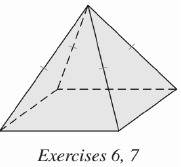Chapter 9.CT, Problem 7CTElementary Geometry For College St...

7th Edition
Alexander + 2 others
ISBN: 9781337614085

Solutions

Chapter
SectionElementary Geometry For College St...

7th Edition
Alexander + 2 others
ISBN: 9781337614085
Textbook Problem

Find the volume of the regular square pyramid shown if each edge of the base measures 5 ft and the altitude measures 6 ft.To determine

To find:

The volume of the regular square pyramid.

Explanation

Given:

For a regular square pyramid with each edge of the base measures 5 ft. and the altitude measures is 6 ft.

Formula used:

The volume of the pyramid is V=13Bh.

Calculation:

Since the base is a square with each edge measuring 5 ft, the base area is

B=55=25 ft2

Still sussing out bartleby?

Check out a sample textbook solution.

See a sample solution

The Solution to Your Study Problems

Bartleby provides explanations to thousands of textbook problems written by our experts, many with advanced degrees!

Get Started

2. Solve for y:

Mathematical Applications for the Management, Life, and Social Sciences

In Exercises 11-16, find the slope of the line that passes through each pair of points. 14. (2, 2) and (4, 4)

Applied Calculus for the Managerial, Life, and Social Sciences: A Brief Approach

In Exercises 1-6, solve for y. x3y+2y=1

Calculus: An Applied Approach (MindTap Course List)